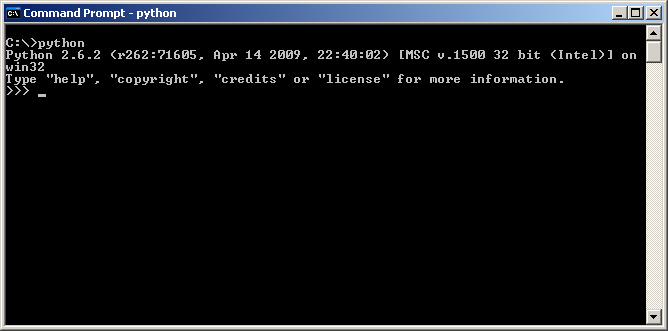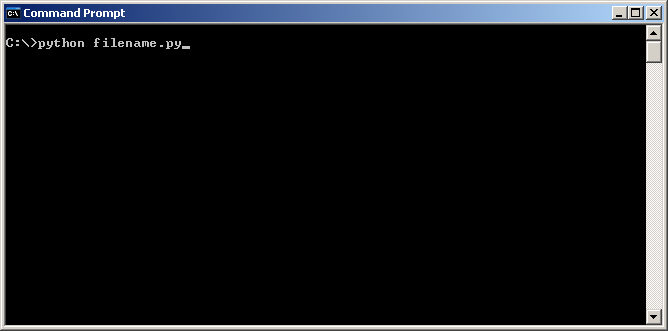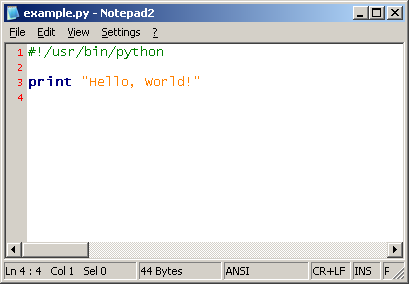# Introduction to Python

### Introduction

This tutorial will introduce you to some of the basic concepts of Python, including syntax, data types, and language constructs. No previous experience with Python is assumed.

### Obtaining and Installing Python

Python is a free and open-source language and can be downloaded for your specific platform at the following URL:

For detailed instructions on installing Python on your particular platform, consult the Python documentation.

Once you have Python installed, you should be able to bring up a command prompt (Windows) or a terminal (UNIX/Linux) and type `python` to start the Python interpreter:The interpreter will evaluate any expression you type in. Alternatively, you may specify a Python script (.py) to be executed:### Writing Python Scripts

Any basic text editor will work fine for writing Python code, although you may want to use an editor with syntax highlighting support for the language, as well as one with the option to indent using spaces rather than tabs.### Python's Syntax: Indentation is King

In Python, blocks of code are distinguished by indentation. This is quite different than C-like languages such as PHP and Javascript, which use curly braces ("{" and "}") to signify the beginning and end of a block of code, respectively. This may feel strange at first, but you'll get used to it, and it can make Python code significantly easier to read.

Here's an example of Python code containing nested code blocks. Notice how the indentation of each line determines which block it belongs to:

``````def foo():
print "Hello, world!"
num = 5
if( num <= 5 ):
temp = num
while( temp > 0 ):
print "The number is", temp
temp -= 1
else:
print "The number is greater than five."
``````

### Basic Keywords and Constructs

Python has two different comment styles that can be used.

The pound symbol style (#) is used to signify a comment that extends through to the end of the line.

``````foo = 5   #this is a comment
bar = 24
#this is also a comment
baz = "hello"
``````

The slash-star style (/ ... /) specifies a comment that begins with a slash-star (/) and ends at the first star-slash (/). Comments of this style can span multiple lines.

``````foo = 5    /*this is a comment*/
bar = 24
/*this comment
spans multiple
lines */
baz = "hello"
``````

Line Endings
No semicolon is needed at the end of a statement in Python; the end of a line implies the end of the statement

``````foo = 5**;**   #wrong!
bar = 42    #correct
``````

Conditionals and Loops
Conditional blocks (if-statements) and loops begin with their respective keywords, an open-parenthesis, the conditions, a closing-parenthesis, and a colon:

``````if( foo > 5 ):     #note the colon!
#these indented lines are part of the conditional
bar = 42
print "hello"
elif( foo < 5 ):               #note that it's "elif", not "else if"
bar = 10
print "hola"
else:
print "bonjour"
``````
``````while( foo > 5 ):     #again, note the colon!
#once again, indentation determines
#the statements within the loop
print "foo is", foo
foo -= 1
``````

### Variables and Basic Data Types

You've already seen some variables used in this tutorial. To define a variable, simple write the name of the variable and set it equal to some value:

``````my_variable = "this is some text"
``````

Python's basic data types include those found in most other languages: integers, floating point numbers, booleans, and strings.

``````my_int_var = 5
my_float_var = 2.467
my_bool_var = True
my_string_var = "a string of text"
``````

### Functions and Classes

Functions
To define a function in Python, we use the def keyword, followed by the name of the function, an open-parenthesis, any parameters for the function, a closing-parenthesis, and a colon:

``````def doSomething(num):
print "The number is", num
``````

Classes
Python is an object-oriented language and thus allows the creation of new types (classes). A class is defined with the class keyword, followed by the name of the class and a colon:

``````class Foo:
some_variable = 5
some_other_variable = "some text"

def doSomething(self, num):
print "The number is", num
``````

We'll explore Python classes further in future tutorials.

### Conclusion

At this point you should have a basic enough understanding of Python to begin writing a few simple scripts. Here are a few sample problems to solve using Python:

• Create a function that prints out all even numbers between 1 and 100
• Modify this function to take an argument specifying the upper end of the range rather than setting it to 100

• Create a function that concatenates two strings together and returns the result

• Create a function that prints out all Fibonacci numbers up to a specified number